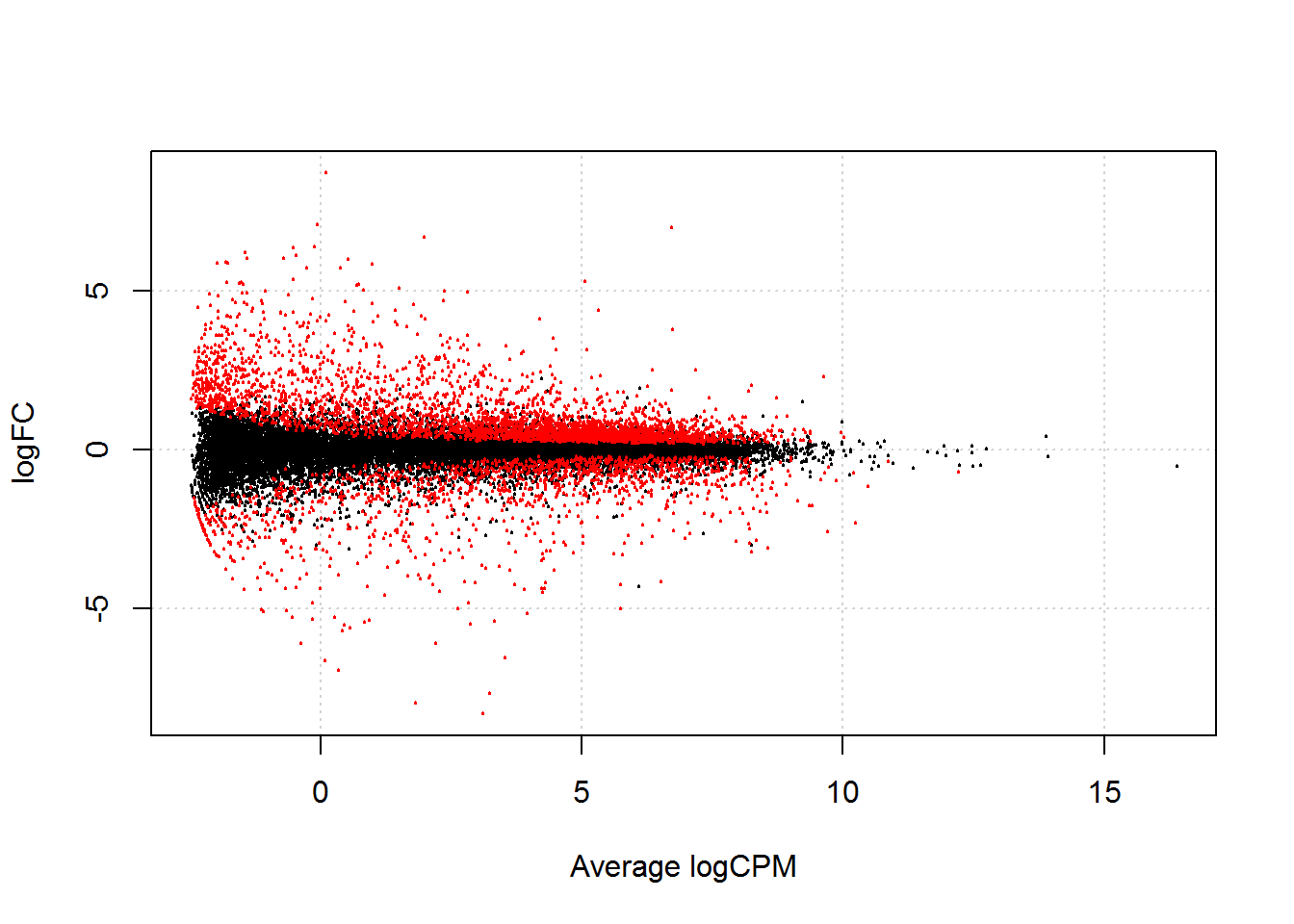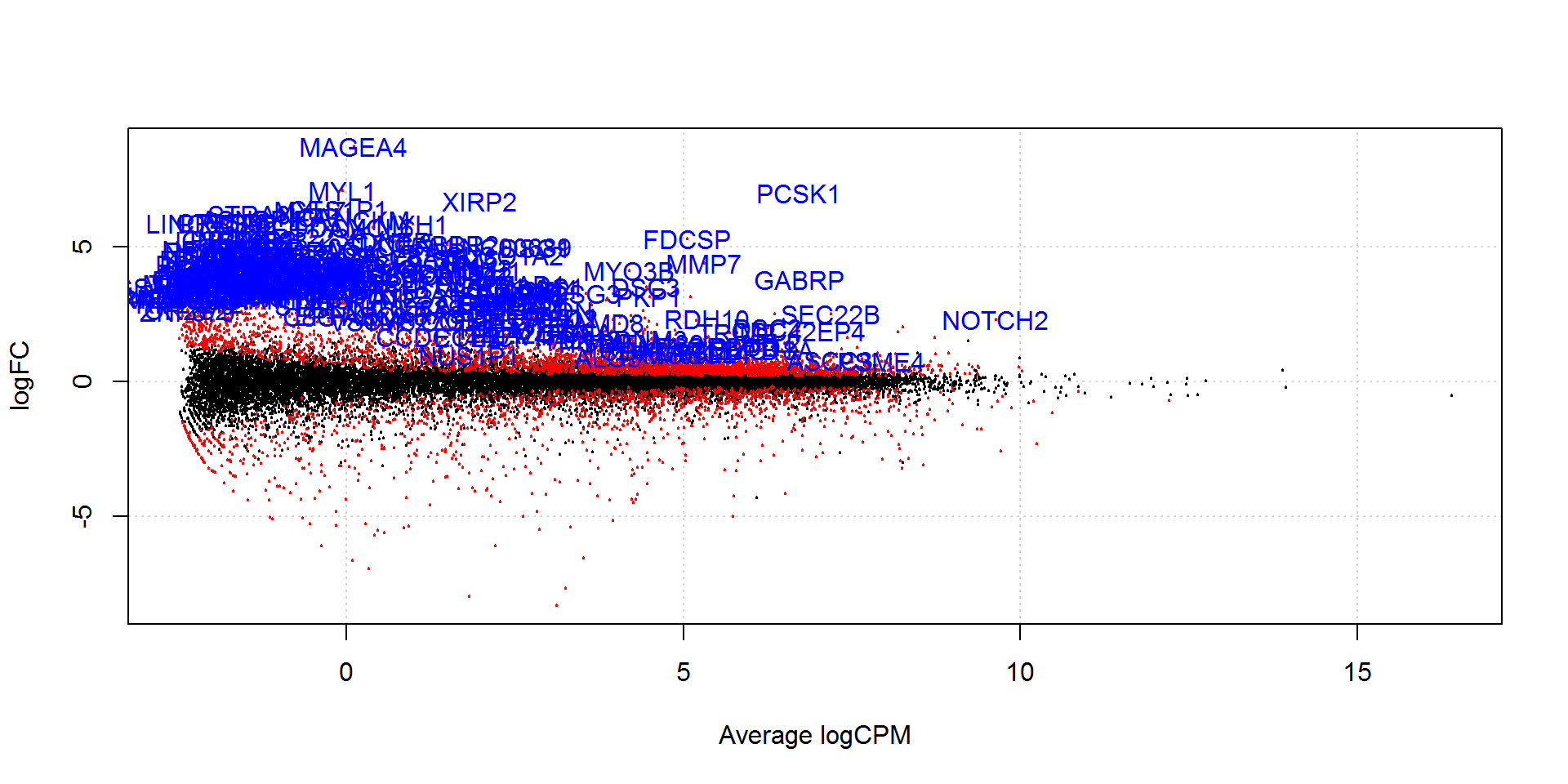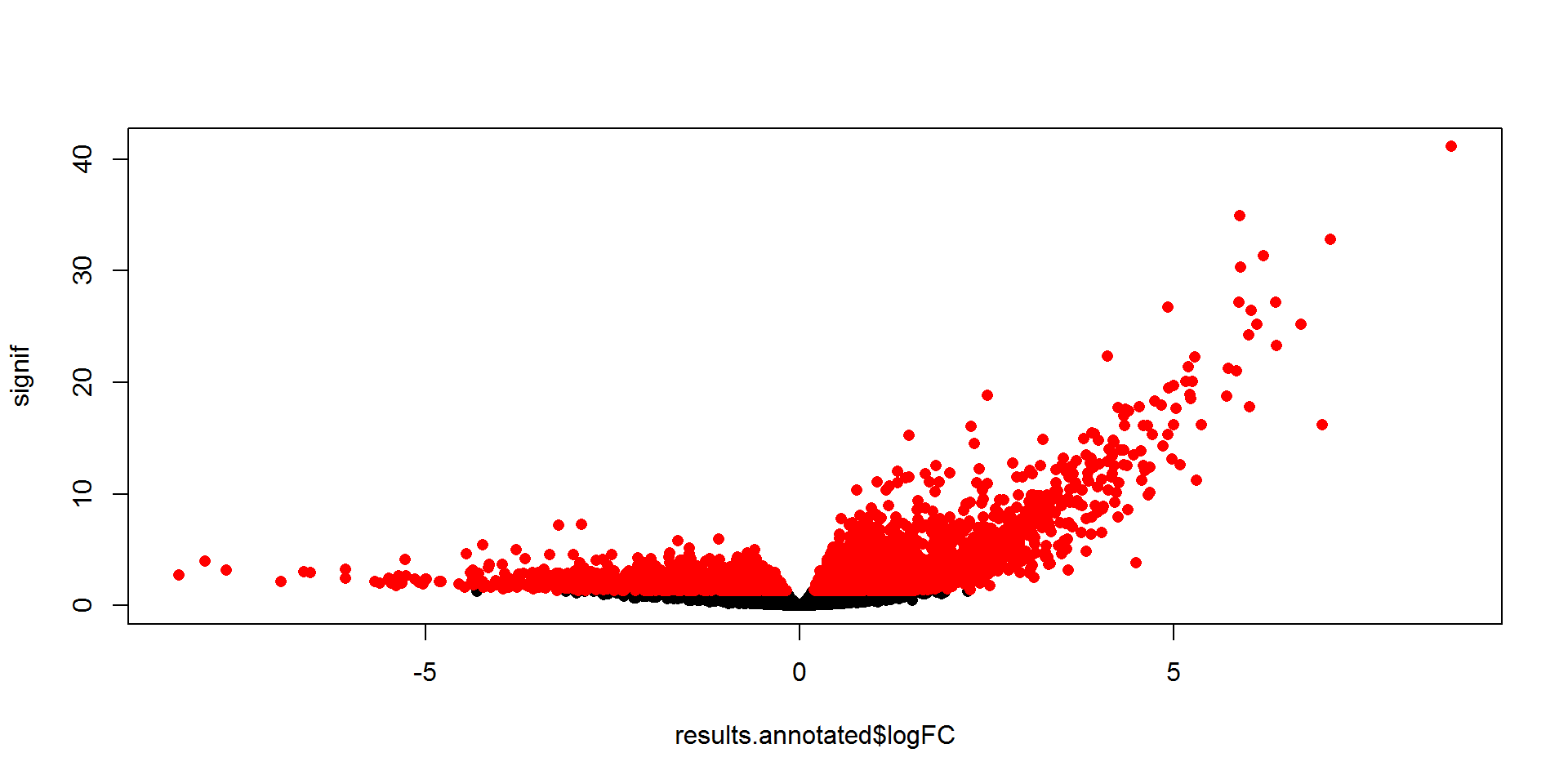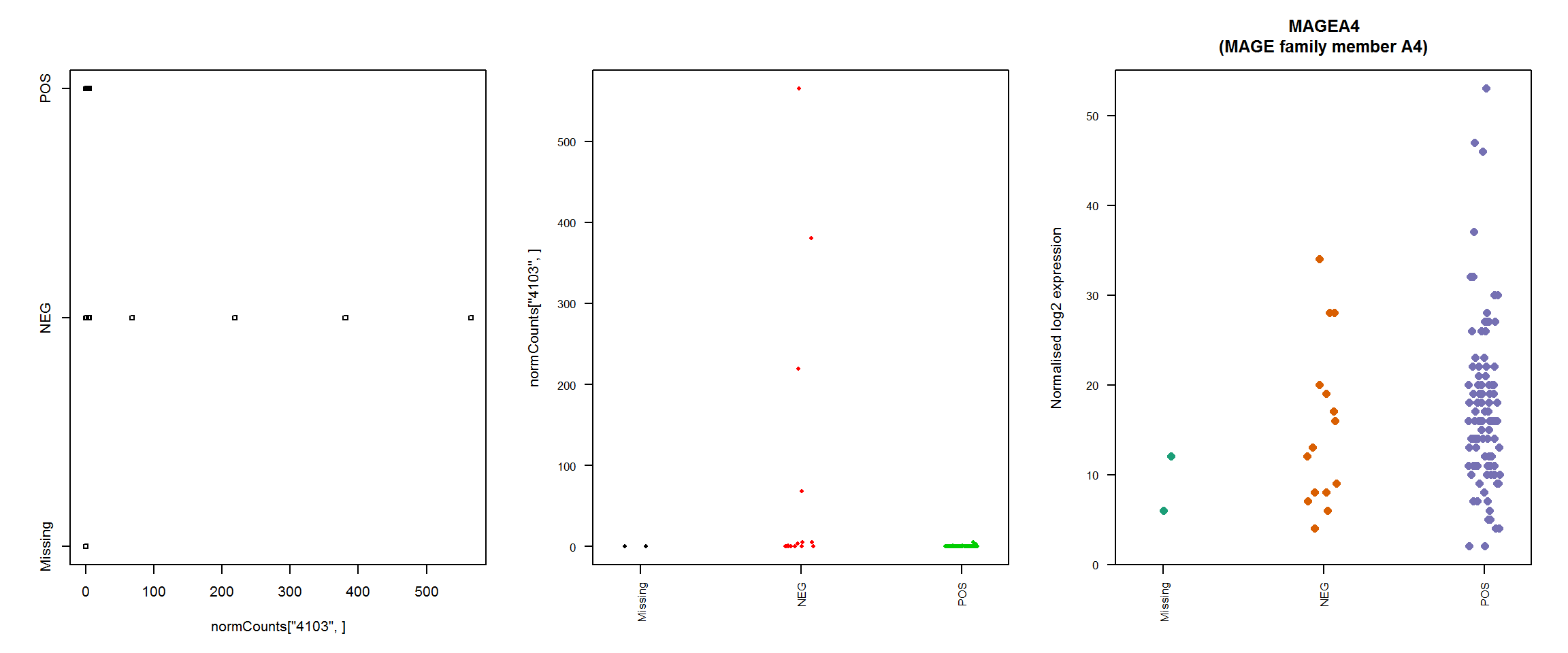``````setwd("C:/Users/chaadams/Dropbox/COH/Hispanic tumours/MD ER Results")
suppressPackageStartupMessages(library(edgeR))

# Overview

• Visualising DE results
• Getting annotation
• Alternate visualizing with ggplots
``````results <- as.data.frame(topTags(res,n = Inf))
dim(results)``````
``##  22356   131``

`edgeR` provides a function `plotSmear` that allows us to visualise the results of a DE analysis. In a similar manner to the MA-plot for microarray data, this plot shows the log-fold change against log-counts per million, with DE genes highlighted:

``summary(de <- decideTestsDGE(res))``
``````##        1*NEG -1*POS
## Down           1424
## NotSig        17593
## Up             3339``````
``````detags <- rownames(dgeObj)[as.logical(de)]
plotSmear(res, de.tags=detags)``````## Adding annotation to the edgeR results

There are a number of ways to add annotation, but I did this using the org.Hs.eg.db package. (I used `biomaRt` to filter our data initially, as it came with ENSEMBL IDs. The pipeline we are using here uses ENTREZ IDs. I selected the genes for which there were unique ENTREZ IDs matched to ENSEMBL IDs in `biomaRt`.)

``````source("http://www.bioconductor.org/biocLite.R")
# For Human
biocLite("org.Hs.eg.db")
library(org.Hs.eg.db)``````
``library(org.Hs.eg.db)``

I got the gene symbols and full gene names.

``ann <- select(org.Hs.eg.db,keys=rownames(results),columns=c("ENTREZID","SYMBOL","GENENAME"))``
``## 'select()' returned 1:1 mapping between keys and columns``
``````# Have a look at the annotation
``````##    ENTREZID    SYMBOL                                   GENENAME
## 1      4103    MAGEA4                      MAGE family member A4
## 2 100874155 LINC00392 long intergenic non-protein coding RNA 392``````

I double checked that the `ENTREZID` column matched exactly to our `results` rownames.

``table(ann\$ENTREZID==rownames(results)) ``
``````##
##  TRUE
## 22356``````

I bound the annotation information to the `results` data frame.

``````results.annotated <- cbind(results, ann)
``````##  "MAGE family member A4"
##  "long intergenic non-protein coding RNA 392"``````

I saved results table using the `write.csv` function.

``write.csv(results.annotated,file="Annotated_ER_Results.csv",row.names=FALSE)``

A note about deciding how many genes are significant: `decideTests` function displays significant genes at 5% FDR.The volcano plot displays a measure of significance on the y-axis and fold-change on the x-axis.

``````signif <- -log10(results.annotated\$FDR)
plot(results.annotated\$logFC,signif,pch=16)
points(results.annotated[detags,"logFC"],-log10(results.annotated[detags,"FDR"]),pch=16,col="red")``````Here is an example sanity check to have a look at the expression levels of the individual samples for a genes of interest. I looked at grouped expression using `stripchart`. I used the normalised log expression values in the `dgeCounts` object (`dgeCounts\$counts`).

``````library(RColorBrewer)
par(mfrow=c(1,3))
normCounts <- dgeObj\$counts
# Let's look at the most signficiant gene, MAGEA4, which has a rowname 4103
stripchart(normCounts["4103",]~groupER)
# This plot is ugly, let's make it better
stripchart(normCounts["4103",]~groupER,vertical=TRUE,las=2,cex.axis=0.8,pch=16,col=1:6,method="jitter")
# Let's use nicer colours
nice.col <- brewer.pal(6,name="Dark2")
stripchart(normCounts["1",]~groupER,vertical=TRUE,las=2,cex.axis=0.8,pch=16,cex=1.3,col=nice.col,method="jitter",ylab="Normalised log2 expression",main="   MAGEA4\n(MAGE family member A4)")``````## More MD plots

MD plot showing the log-fold change and average abundance of each gene. Signficantly up and down DE genes are highlighted in red and blue respectively.

``````library(org.Hs.eg.db)
library(statmod)
design <- model.matrix(~0+groupER)
colnames(design) <- levels(groupER)
y <- DGEList(counts.keep,group=groupER, genes=counts.keep)
options(digits=3)

# Add the gene names to the DGEList object
y\$genes\$Symbol <- rownames(counts.keep)
y <- estimateDisp(y,design, robust=TRUE)

## estimation of QL dispersions
fit <- glmQLFit(y, design, robust=TRUE)
``````##           Missing    NEG    POS
``plotQLDisp(fit)``